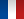Design & Organisation
High level education# Mathematics and Computer Science for Decision Support - 3GUC0905

A+Augmenter la taille du texteA-Réduire la taille du texteImprimer le documentEnvoyer cette page par mail Partagez cet article Facebook Twitter Linked In
• #### Number of hours

• Lectures : 24.0
• Tutorials : 18.0
• Laboratory works : 33.0
• Projects : -
• Internship : -
• Written tests : 4.0
ECTS : 6.0
• Officials : Olivier BRIANT

### Goals

Objectives of these lessons are to give students learning in the elementary decision tools based in mathematics and informatics. Lessons are constituted of three courses :
Statistics to establish preliminary understanding of problems through data analysis and to support decision facing risky situation ; Linear programming to model and solve problem under constraints ; and Computer science as set of tools useful to manage, analyze and solve data and problems.

Statistics provide models of random situation (based on Probability) and tools to specify risk and to decide facing random environment. Applications come from quality
management, production monitoring, market and marketing studies. Lessons topics are : descriptive statistics - to summarize and to represent data ; inferential statistics
(introduction to estimation and test theory) - to extract knowledge from survey and/or experimental data and to help decision and prediction.

Linear programming as operations research tool allows to model complex problems by identifying variables (continuous or integer), by modeling the problem constraints as equations and linear inequalities on these variables, and by expressing quality of the solutions using a linear function. The study of the solutions produced by the simplex algorithm, coupled with the notion of duality allows a sensitivity analysis of the solutions very useful in decision making. In integer linear programming, the Branch and Bound algorithm will be studied, as well as the concept of quality modeling to improve the resolution.

The use of Computer science as a tool for modeling and solving is enhanced during an IT project for a period equivalent to two full weeks. The students, in teams, shall propose a IT solution at a given industrial problem.

Students will gain skills in:

• Statistics :
• handling data and statistical tools and concepts : descriptive statistics, estimator, confidence interval, linear regression
• building test and decision based on statistical risk evaluation : to test hypothesis and to decide
• using software to simulate, analyze data and to build test hypothesis (Excel, R)
• Linear Programming:
• modeling a simple problem using a linear program, including translating the constraints of the problem through a system of linear equations or inequalities
• solving the program using the simplex algorithm and giving different economic interpretations of the output of this algorithm, using the duality theory
• using linear solvers such as Excel or ILOG
• Computer science :
• analyzing a problem and identifying the concepts involved
• developing in Java a clean and modular software
• using Eclipse development software
• working in a team

Content

• Statistics : 2 x 1,5h lessons, 7 x 3h lessons/application
• Descriptive statistics
• Estimator and confidence interval
• Introduction to statistical hypothesis test (test of the mean (one-sample z-test), test of proportion (one-proportion z-test), chi-square test for goodness-of-fit or for independence
• Linear programming : 7 x 1,5h lessons, 7 x 1,5h application, 2 x 3h use of software
• Modelling a problem in a linear program
• Resolution algorithms: geometrics, simplex and variants
• Duality
• Sensitivity analysis
• Notions of integer linear programming
• Branch & Bound algorithm
• Quality of the various modellisations
• IT Project
• First, students must analyze the problem, understand the difficulties, and appropriate it to structure the data, to understand the concepts involved, and propose appropriate solution methods.
• In a second full week, each pair of students will draw up their chosen solution in Java Language. Both the quality of the solution found and the quality of the IT skills must be taken into account.
• This computer project is to be handed in at the end of the second week and is assessed on various different criteria:
• relevance of the choice of data structure
• the quality of the top-down analysis
• the robustness of the program and the solution methods
• quality of the solutions found

Prerequisites

• Basic courses in mathematical analysis and probability.
• Basic courses in linear algebra and matrices.
• IT course from Semester 1

Tests

IT Project (P) : report and computer programms

E1 = Final written exam from 1st exam period - duration 4 hours
E2 = Written exam from 2nd exam period - duration 4 hours

N1 = 0.7*E1 + 0.3*P
N2 = E2

Calendar

The course exists in the following branches:

• Curriculum - Engineer student Bachelor - Semester 6
see the course schedule for 2019-2020

Course ID : 3GUC0905
Course language(s):You can find this course among all other courses.

Bibliography

Tous ces livres sont disponibles à la bibliothèque de Grenoble INP - Génie industriel :

• Statistique, Wonnacott and Wonnacott, 1998.
• Introductory Statistics, Wonnacott and Wonnacott
• Statistique appliquée à la gestion, Vincent Giard
• http://www.math-info.univ-paris5.fr/~smel/ : ce site propose un cours, des jeux de données, des articles de réflexion, et surtout beaucoup d'applications pour visualiser, tester et comprendre les différents concepts du cours
• Linear Programming, Vasek Chvatal, Freeman & co, 1983, ISBN 0716715872
• Programmation Linéaire, Christelle Guéret, Christian Prins et Marc Sevaux, Eyrolles, 2000, ISBN 2-212-09202-4
• Fascicule de cours d'informatique d'Hadrien Cambazard du semestre 1

A+Augmenter la taille du texteA-Réduire la taille du texteImprimer le documentEnvoyer cette page par mail Partagez cet article Facebook Twitter Linked In

Date of update June 5, 2015